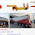Now we will start here, in this post, another important topic i.e. deflection and slope of a cantilever beam loaded with uniformly distributed load throughout the length of the beam with the help of this post.

We have already seen terminologies and various terms used in deflection of beam with the help of recent posts and now we will be interested here to calculate the deflection and slope of a cantilever beam loaded with uniformly distributed load throughout the length of the beam with the help of this post.

Cantilever beam is basically defined as a beam where one end of beam will be fixed and other end of beam will be free.

Uniformly distributed load is the load which will be distributed over the length of the beam in such a way that rate of loading will be uniform throughout the distribution length of the beam

Basic concepts

There are basically three important methods by which we can easily determine the deflection and slope at any section of a loaded beam.
Double integration method
Moment area method
Macaulay’s method

Double integration method and Moment area method are basically used to determine deflection and slope at any section of a loaded beam when beam will be loaded with a single load.

While Macaulay’s method is basically used to determine deflection and slope at any section of a loaded beam when beam will be loaded with multiple loads.

We will use double integration method here to determine the deflection and slope of a cantilever beam which is loaded with uniformly distributed load throughout the length of the beam.

Differential equation for elastic curve of a beam will be used in double integration method to determine the deflection and slope of the loaded beam and hence we must have to recall here the differential equation for elastic curve of a beam.

Differential equation for elastic curve of a beam

After first integration of differential equation, we will have value of slope i.e. dy/dx. Similarly after second integration of differential equation, we will have value of deflection i.e. y.

Let us come to the main subject i.e. determination of deflection and slope of a cantilever beam which is loaded with uniformly distributed load throughout the length of the beam.

Let us consider a cantilever beam AB of length L which is fixed at the support A and free at point B and loaded with uniformly distributed load as displayed in following figure.
We have following information from above figure,
θA = Slope at support A
θB = Slope at support B
yB = Deflection at free end B
Total load due to udl = W = w.L

Boundary condition

We must be aware with the boundary conditions applicable in such a problem where beam will be a cantilever beam with udl. We have following boundary condition as mentioned here.

At point A, Deflection will be zero
At point A, Slope will be zero
At point B, Deflection will be maximum
At point B, Slope will also be maximum

Let us consider one section XX at a distance x from end support A, let us calculate the bending moment about this section.
We have taken the concept of sign convention to provide the suitable sign for above calculated bending moment about section XX. For more detailed information about the sign convention used for bending moment, we request you to please find the post “Sign conventions for bending moment and shear force”.
Let us consider the bending moment determined earlier about the section XX and bending moment expression at any section of beam. We will have following equation as displayed here in following figure.
We will now integrate this equation and also we will apply the boundary conditions in order to secure the expressions for slope as well as deflection at a section of the beam and we can write the equations for slope and deflection for loaded beam as displayed here.
Where, C1 and C2 are the constant of integration and we can secure the value of these constant C1 and C2 by considering and applying the boundary condition.

Let us use the boundary condition as we have seen above.
At point A i.e. x = 0, Slope will be zero i.e. dy/dx =0
At point A i.e. x = 0, deflection will be zero i.e. y = 0

After applying the boundary conditions in above equations of slope and deflection of beam, we will have following values of constant C1 and C2 as mentioned here.
C1 = - wL3/6
C2 =  wL4/24

Let us insert the values of C1 and C2 in slope equation and in deflection equation too and we will have the final equation of slope and also equation of deflection at any section of the loaded beam. We can see the slope equation and deflection equation in following figure.

Slope at the free end

At x = L, θB = Slope at end B
Let us use the slope equation and insert the value of x = L, we will have value of slope at support B i.e. θB
θB = - w.L3/6EI
θB = -W.L2/6EI
Negative sign represents that tangent at end B makes an angle with beam axis AB in anti-clockwise direction.

Maximum deflection

At point B i.e. x = L, Deflection will be maximum
Let us use the deflection equation and insert the value of x = L in deflection equation, we will have value of deflection at point B.
yB = - wL4/8EI
yB = - WL3/8EI
Negative sign represents here that deflection in the loaded beam will be in downward direction.
We will see another topic in our next post.

Please comment your feedback and suggestions in comment box provided at the end of this post.

Reference:

Strength of material, By R. K. Bansal
1.